## 神经网络的历史

• 其中一个输入是激活的
• 每个输入的权重相等
• 该模型的输出是二元的

Hebb 在其 1949 年的著作《The Organization of Behaviour（行为组织）》中第一次提出了这一思想：为了应对任务的变化，大脑中的连接会不断发生改变。这样的规则意味着两个神经元之间的连接在这同一时间内是激活的。这一思想很快就变成了开发学习的计算模型和自适应系统的灵感来源。

15 年后，Rosenblatt 于 1958 年开发出了感知器（perceptron），成为了神经元的下一个模型。感知器是最简单的神经网络，能将数据线性地分为两类。后来，他随机地将这些感知器互相连接，并使用了一种试错方法来改变权重以进行学习。

1969 年，在数学家 Marvin Minsky 和 Seymour Parpert 发表了一篇对感知器的数学分析后，这个方向的研究在接下来的 15 年里陷入了停滞。他们的研究发现感知器无法表征很多重要的问题，比如异或函数（XOR）。其实，那时的计算机还没有足够的处理能力来有效地处理大型神经网络。

1986 年，Rumelhart、Hinton 和 Williams 报告他们所开发的反向传播（back-propagation）算法能够解决 XOR 这样的问题，并由此开启了第二代神经网络。同一年，Rumelhart 和 McClelland 编辑的两卷知名著作《Parallel Distributed Processing: Explorations in the Microstructures of Cognition（并行分布式处理：认知的微结构中的探索）》发表。这本书在反向传播的应用上产生了很大的影响，而反向传播也已经在多层感知器的训练上成为了最为流行的学习算法。

## 单层感知器（SLP）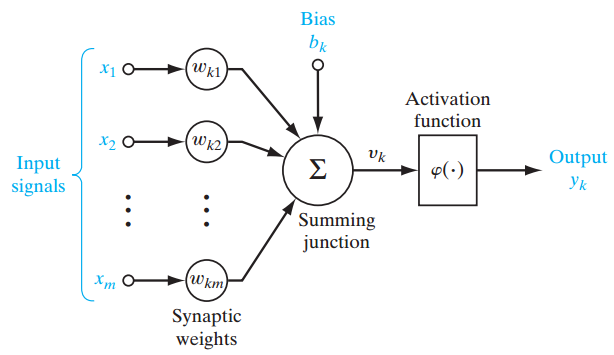$$u_{k} = {\sum _ {j=1}^m} w_{kj}x_j$$

$$v_{k} = u_{k} + b_{k}$$

## 多层感知器（MLP）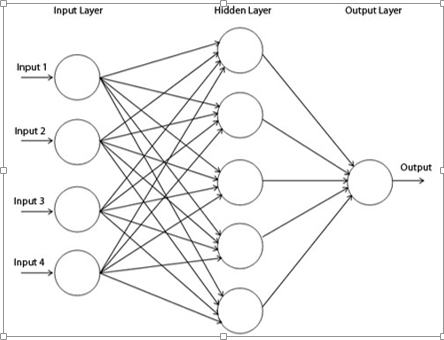• 参数的初始化

$$\left\lfloor -\sqrt{\frac 6{fan_{in}+fan_{out}}}, \sqrt{\frac 6{fan_{in}+fan_{out}}} \right\rfloor$$

$$\left\lfloor -\sqrt{\frac 6{fan_{in}+fan_{out}}}, \sqrt{\frac 6{fan_{in}+fan_{out}}} \right\rfloor$$

• 激活函数

$$y_{k} = \varphi(u_{k} + b_{k})$$

$$\varphi_{(v)}=\begin{cases} 1\quad if\quad v\ge0 \\ 0\quad if\quad v\lt0 \end{cases}$$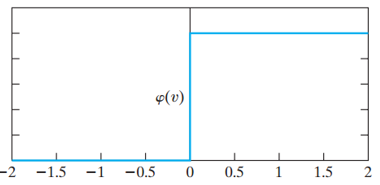1.阈值函数（Threshold Function）

1. S 型函数（Sigmoid Function）

$$\varphi_{(v)}=\frac{1}{1+exp^{(-av)}}$$

3.双曲正切函数（Hyperbolic Tangent Function）

φ(v) = tanh (v)

4.修正线性激活函数（ReLU）

ReLU 是许多逻辑单元之和的平滑近似，能够产生稀疏的活动向量。下面是该函数的方程式：
$$y=max\left\lbrace\begin{matrix} 0,b+\sum_{i=1}^k {x_i \ast w_i} \\ \end{matrix}\right\rbrace$$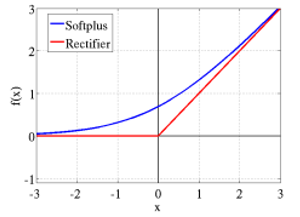$$(softplus \quad f(x)=log(1+e^x)$$

5. Maxout 函数

2013 年，Goodfellow 发现使用了一种新的激活函数的 Maxout 网络是 dropout 的天然搭档。

Maxout 单元能促进 dropout 的优化以及提升 dropout 的快速近似模型平均技术（fast approximate model averaging technique）的准确度。单个 Maxout 单元可被理解为是对一个任意凸函数的片（piece）形式的线性近似。

Maxout 网络不仅能学习隐藏单元之间的关系，还能学习每个隐藏单元的激活函数。下面是关于其工作方式的图形化描述：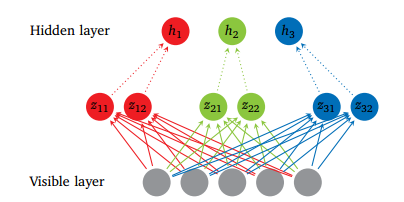$$h_i=max_{je[1,k]}z_{ij}$$
$$z_{ij}=\sum_m x_mW_{mij}+b_{ij}=xW_{\ldots ij}+b_{ij}$$

$$W \in \mathbb R^{m \times n \times k}$$

- 反向传播算法

1.mini-batch 梯度下降

2.随机梯度下降

3.full batch 梯度下降

- 成本函数（Cost Function）

1.均方误差函数
$$E = \frac 12 \sum_{i \in Output}(y_i-o_i)^2$$

2.交叉熵函数
$$-\frac 1n \sum_{i=1}^n log(f(x_i,l_j,W))$$

3.负对数似然损失（NLL）函数

NLL 是用于本报告的所有实验中的成本函数：
$$NLL(0,D)=-\sum_{i=0}^{|D|}log P(Y=y^{(i)}|x^{(i)},\theta)$$

- 学习率（Learning rate）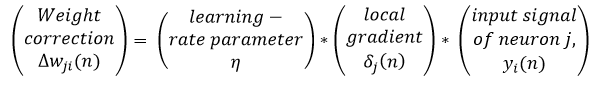• 动量（Momentum）
动量为避免局部极小值提供了惯性；其思想简单来说就是为当前的权重更新增加特定比例的先前的权重更新，这有助于避免受困于局部极小值。
$$\Delta w_{ij}(n)=a\Delta w_{ji}(n-1)+\eta \delta_j(n)y_i(n)$$
其中 α是动量。

• Softmax
Softmax 是一种神经传递函数，其是可将向量变成概率的输出层中实现的 logistic 函数的广义形式。这些概率的总和为 1 且限定于 1。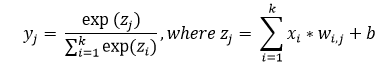• 多层感知器（MLP）：总结

## 深度学习概览

Bengio 认为隐藏层中的神经元可被看作是其下面的层中的神经元所学到的特征检测器（feature detector）。这个结果处于作为一个神经元子集的更好泛化（generalization）中，而这个神经元子集可从输入空间中的特定区域的数据上进行学习。

### 受限玻尔兹曼机和深度信念网络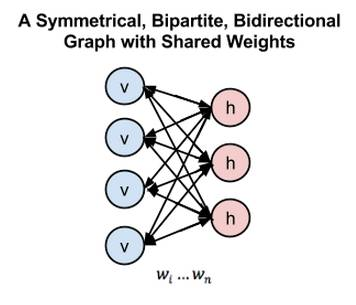RBM 的权重是被随机初始化的，造成了分布中 p(x) 和 q(x) 的不同。在学习过程中，权重被迭代调整，从而最小化 p(x) 和 q(x) 之间的误差。在图 2 中，q(x)是源数据的近似，p(x) 是源数据。

### DROPOUT

Dropout 是 Hinton 引入的一种深度神经网络的正则化技术，通过在每一个训练迭代上随机关掉一部分神经元，而是在测试时间使用整个网络（权重按比例缩小），从而防止特征检测器的共适应。

Dropout 通过等同于训练一个共享权重的指数模型减少过拟合。对于给定的训练迭代，存在不同 dropout 配置的不同指数，所以几乎可以肯定每次训练出的模型都不一样。在测试阶段，使用了所有模型的平均值，作为强大的总体方法。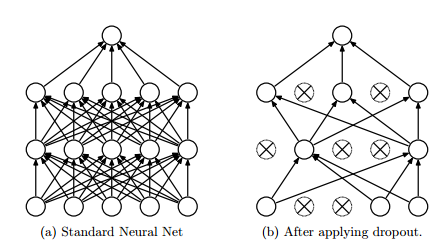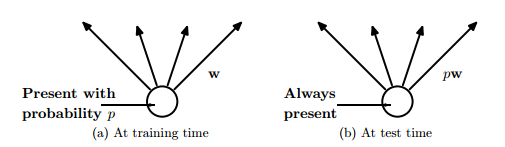Dropout 是平均很多大型神经网络的有效方式。每次训练模型的隐藏单元的时候都能像图 8 中那样以一定的概率漏掉一些。这个概率通常是 ρ=0.5 时。因此，带有减半的开支权重（outgoing weight）的「平均网络」模型可以在测试时间像图 4 中那样使用。平均网络等同于采用概率分布的几何平均数，该分布是通过所有带有单隐层单位和 Softmax 输出层的网络进行标记预测的。

$$P_e(y|x)$$

$$P_d(y|x)$$

d 是区分将哪个输入包括到 softmax 分类器中的二元向量。
$$P_d(y|x) = softmax(W \ast (x \ast d))$$

$$P_d(y|x) = \frac{\prod_dp_d(y|x))^{1/2\^N}}{\sum_{y}\prod_dp_d(y|x))^{1/2\^N}}$$